# How to Use Do While Loop in Excel VBA?

• Last Updated : 30 Jul, 2021

A Do…While loop is used when we want to repeat certain set of statements as long as the condition is true. The condition may be checked at the starting or at the end of the loop

### Flowchart: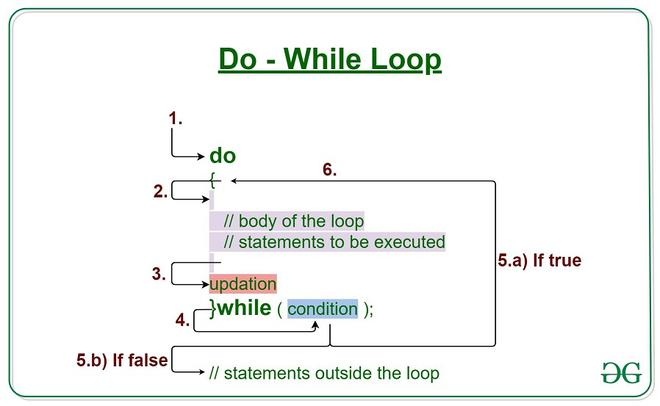### Uses of Do-While loop:

The Do While loop is used in two ways:

• Do…while loop which checks the condition at the STARTING of the loop.
• Do…while loop which checks the condition at the  END of the loop.

Syntax 1:

```Do While condition
[statements]
[Exit Do]
[statements]
Loop  ```

Syntax 2:

```Do While
[statements]
[Exit Do]
[statements]
Loop  condition```

### Implementing a Do While loop:

Follow the below steps to implement a Do-While loop:

Step 1: Define a Macro

```Private Sub Demo_Loop()
End Sub```

Step 2: Define variables

```j=2
i=1```

Step 3: Write Do While Loop. You can write condition at the beginning or at the end

`Do While i < 5`

Step 4: Write statements to be executed in loop

```msgbox "Table of 2 is : " & (j*i)
i=i+1```

Step 5: End loop.

Now let’s take a look at some of the examples.

Example 1: Do…while loop which checks the condition at the STARTING of the loop. The below example uses Do…while loop to check the condition at the starting of the loop. The statements inside the loop are executed, only if the condition is True. We will print Table of 2 using Do…while loop;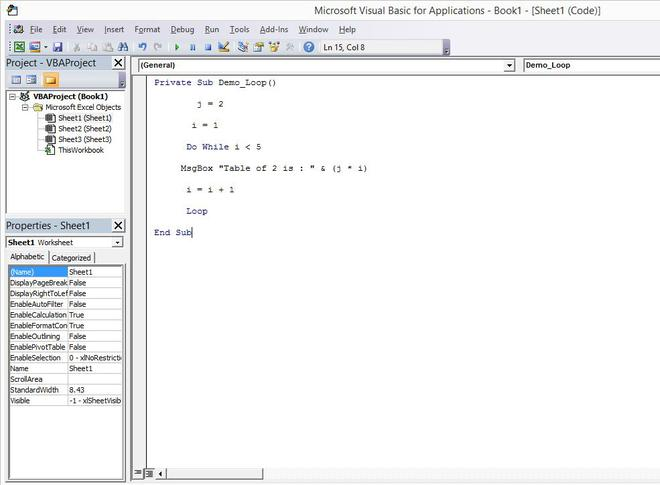```Private Sub Demo_Loop()
j=2
i=1
Do While i < 5
msgbox "Table of 2 is : " & (j*i)
i=i+1
Loop
End Sub```

Output: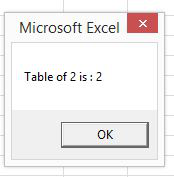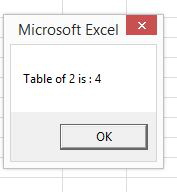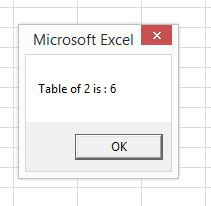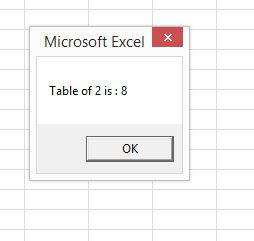Example 2: Do…while loop which checks the condition at the  END of the loop. The below example checks the condition at the end of the loop. The major difference between these two syntax is explained in the following example.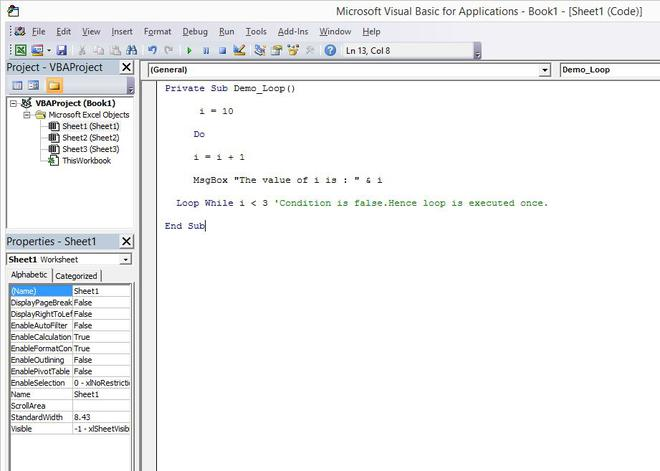```Private Sub Demo_Loop()
i = 10
Do
i = i + 1
MsgBox "The value of i is : " & i
Loop While i < 3 'Condition is false.Hence loop is executed once.
End Sub```

When the above code is executed, it prints the following output in a message box.

Output: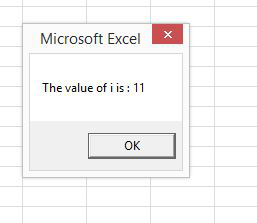My Personal Notes arrow_drop_up
Recommended Articles
Page :